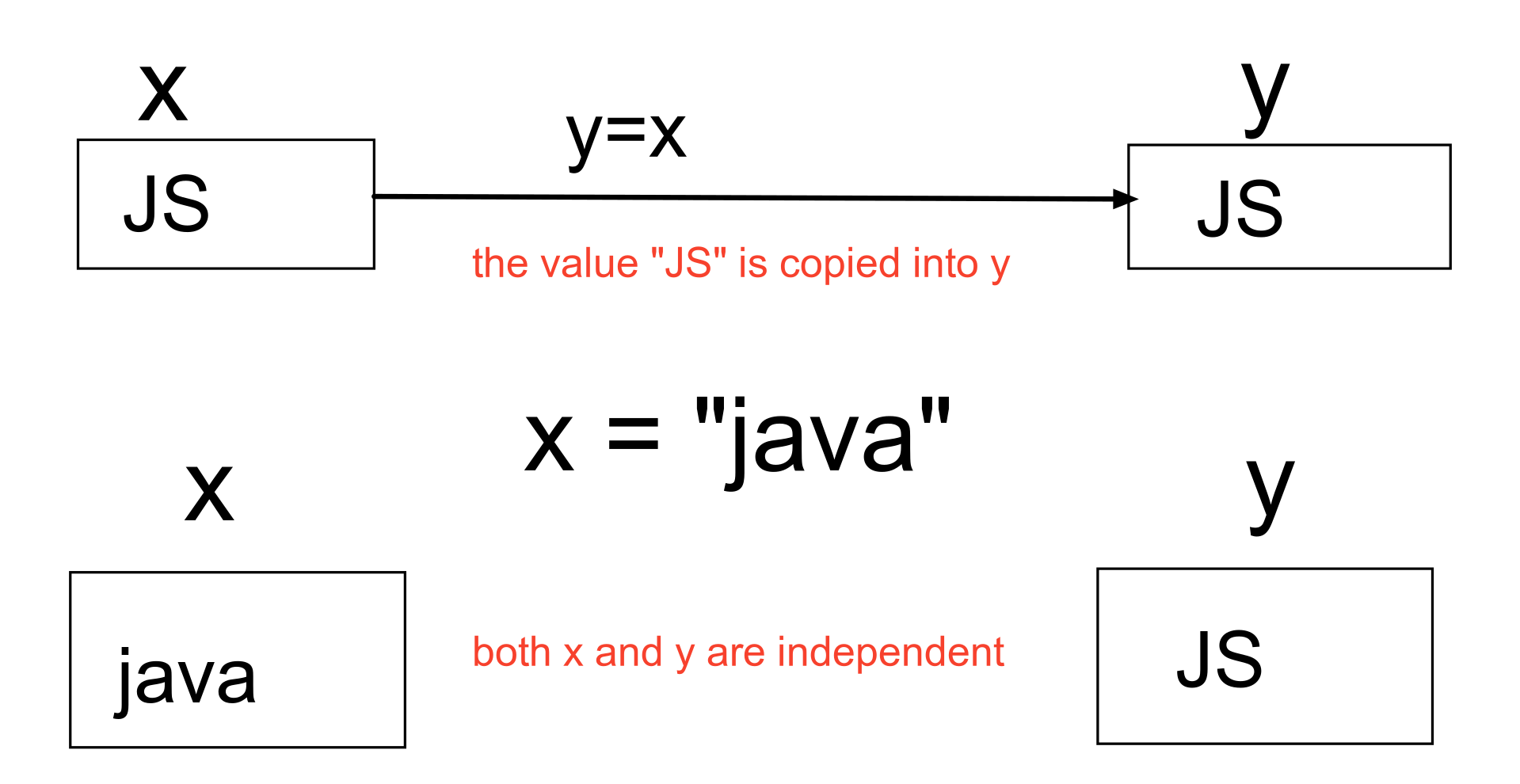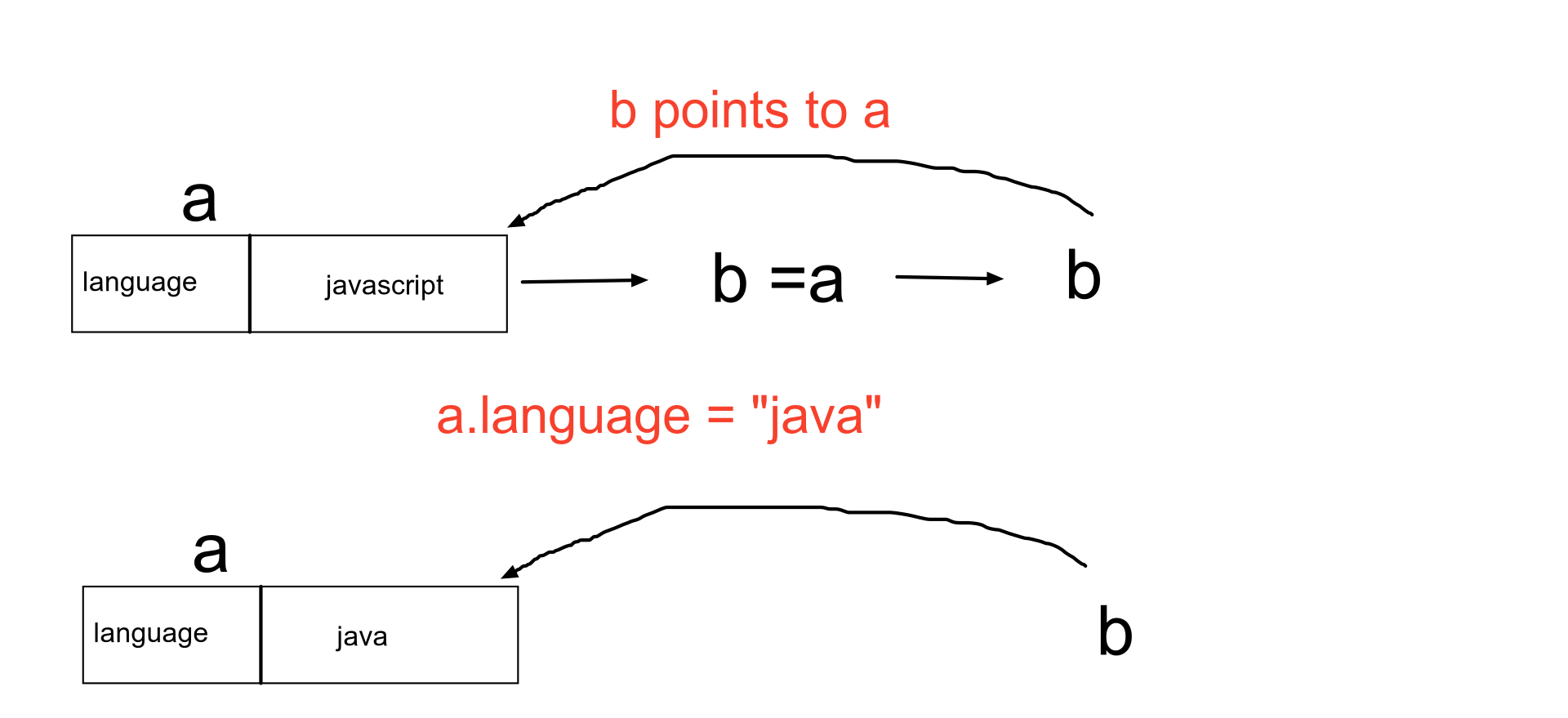.css-4zleql{display:block;}vineethsagarIntroduction to reference types in JavaScript

vineeth sagar
·Nov 18, 2020·

Subscribe to my newsletter and never miss my upcoming articles

In the previous article, we looked into primitive types I would recommend you to read it here before going through this article.

The basic difference between primitive and reference types is that in primitive types the values are stored in the variable whereas in reference types the reference/address to that variable is stored in the variable. Let us understand the difference between both using an example.

Example:

//  primitive

let x = "JS";
let y = x;

console.log(y);  // "JS"

x = "Java";

console.log(x);  // "Java"
console.log(y);  // "JS"

//  reference

let a = {language:"JavaScript"};
let b = a;

console.log(b);  // {language:"JavaScript"}

a.language = "Java";

console.log(a);  // {name:"Java"}
console.log(b);  // {name:"Java"}

primitivereferenceAnother difference between primitive and reference types is that primitive types are stored in the stack whereas reference types are stored in a heap as their size varies dynamically.

In primitive types we use typeof operator to find whether a given datatype is primitive or not, whereas in reference types we use instanceof operator to find whether the given type is a reference type or not.

JavaScript has 3 reference data types, we will understand each one with an example.

Arrays

In JavaScript, if you assign an array to a variable it is the reference to the array that the variable holds not the value so any changes to the array will reflect on the original array lets us look at an example to understand better

Example

let languages = ["c","c++","java"];
let lang = languages;

languages = "javascript";

console.log(lang);  // ["c","c++","javascript"]

Functions

In functions when you pass primitive type data, any changes only happen to formal arguments but doesn't reflect on actual arguments. Let us look at an example.

function foo(val){
val+="script";
}

let lang = "java";
let result = foo(lang);

console.log(lang);  // java
console.log(result); // javascript

In the above example, you can see that changes in the formal arguments are not reflected in actual arguments.

However, in reference types when you can pass an object to a function you can modify its properties but not the object. Look at the example below to understand better

// Example 1
function modifyProperty(obj){
obj.value = 10;
}

let x = {
value : 1;
}

modifyProperty(x);
console.log(x); // { value : 10}

// Example 2
function modifyObject(obj){
obj = {
value = 20;
}
}

ley y = { value: 2 };
modifyObject(y);

console.log(y);  // {value:2}

Objects

In JavaScript, a variable that stores an object is accessed by its reference instead of value.

Refer to the first example to get a better understanding.